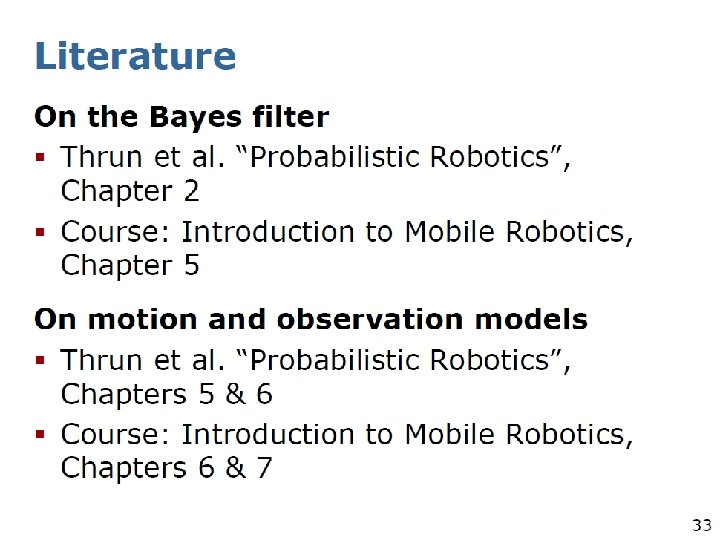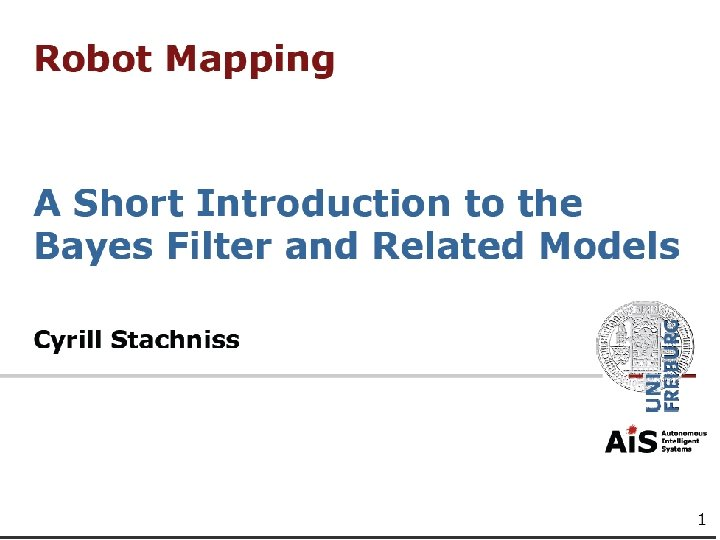# Recursive Bayes Filters and related models for mobile

• Slides: 42
Download presentationRecursive Bayes Filters and related models for mobile robotsRecursive Bayes Filters We will briefly review our derivation of Bayes filter from one of previous lectures first.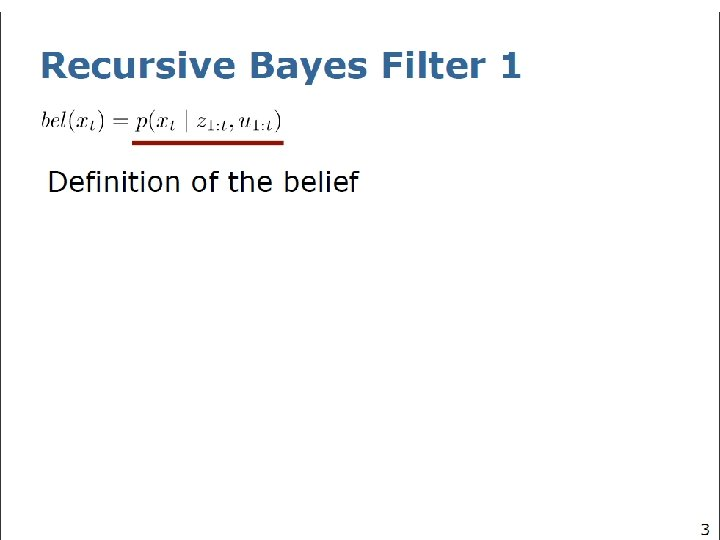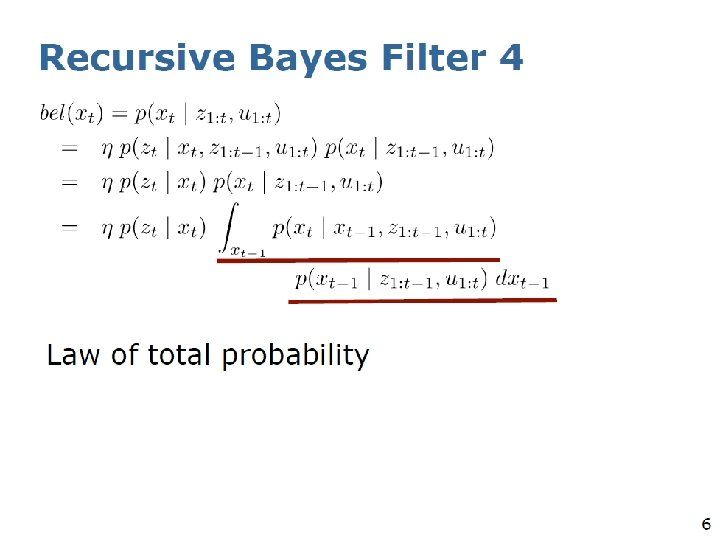Two steps of Bayes filter: Prediction and CorrectionTwo steps of Bayes filter: Prediction and Correction control From odometry and equations of motion • Use measurement to correct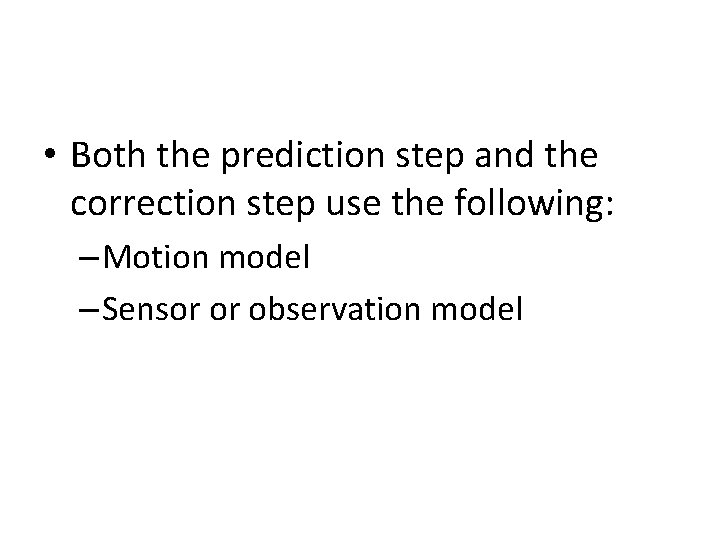• Both the prediction step and the correction step use the following: – Motion model – Sensor or observation model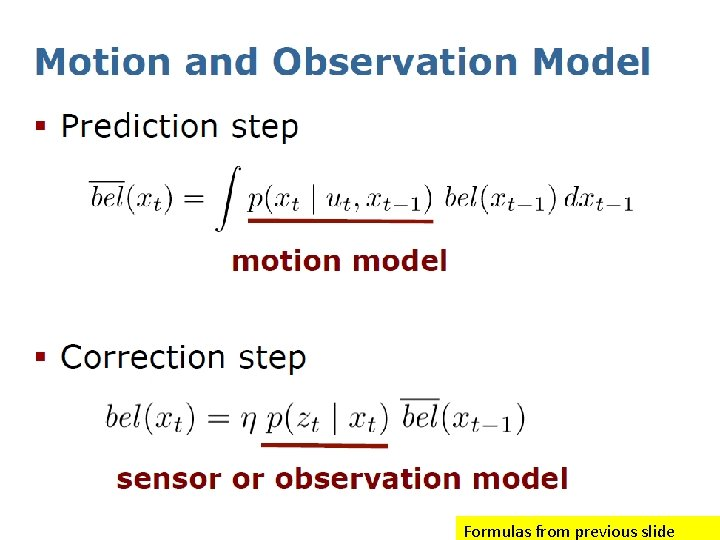Formulas from previous slideDifferent Realizations of Bayes Filters • Recursive filtersMain Approaches to Bayes Filters • Similar methods based on Bayesian probability, networks, and evolutionary algorithms also exist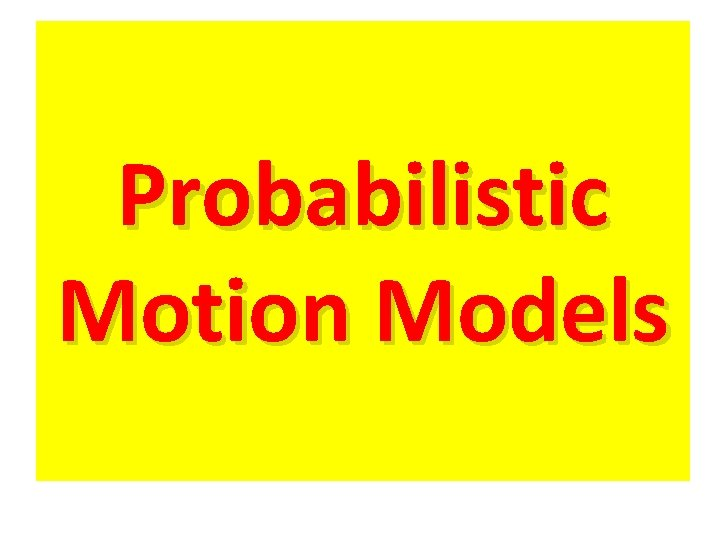Probabilistic Motion Models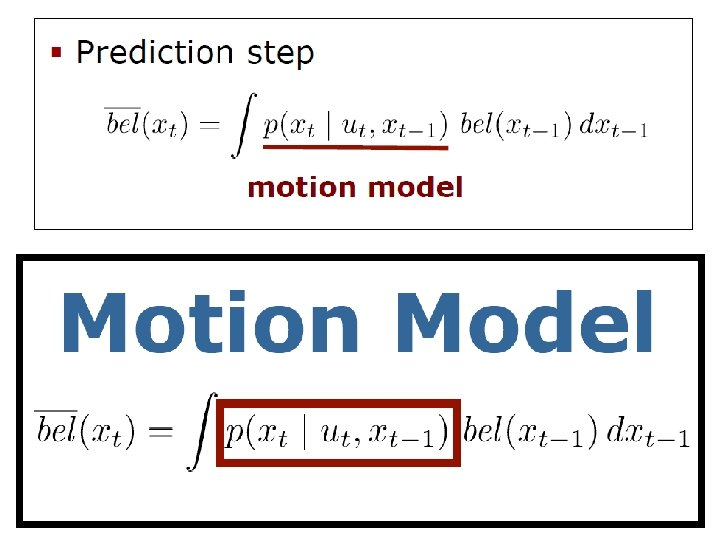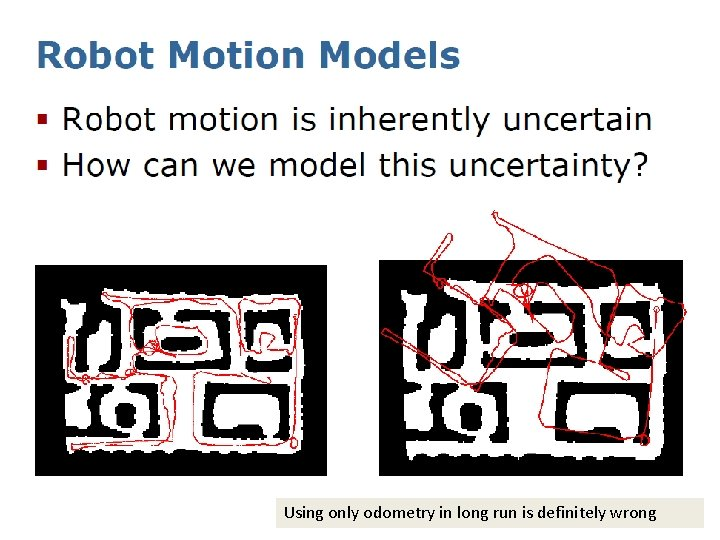Using only odometry in long run is definitely wrongExplain the meaning• In past we used velocity models for simple Braintenberg Vehicles • For MCECSBOT we will have to use perhaps the odometry-based modelMotion Model based on ODOMETRYMotion Model for a robot based on ODOMETRY • This model will be more complicated for OMNI and MECCANO WHEELS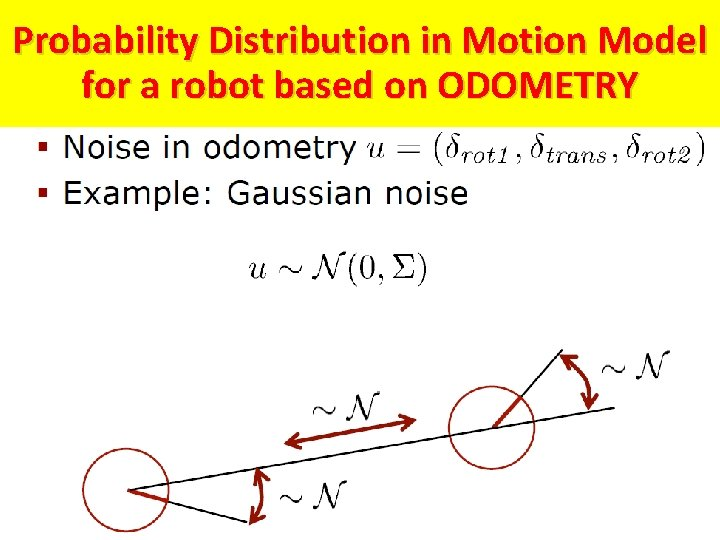Probability Distribution in Motion Model for a robot based on ODOMETRY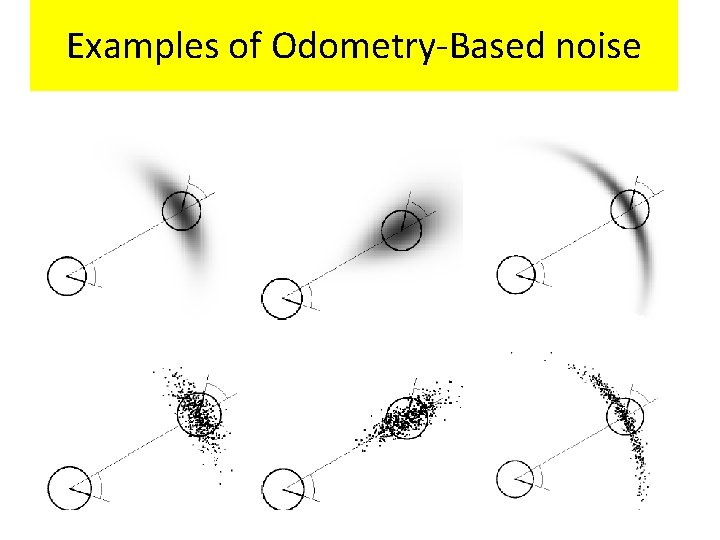Examples of Odometry-Based noiseVelocity Based Motion Models for a robotVelocity Based Motion Models for a robot Explain the meaning • • It is easy to derive such model for a two-wheeled robot We have done it as part of kinematics explanation in Fall quarter (for non-deterministic case).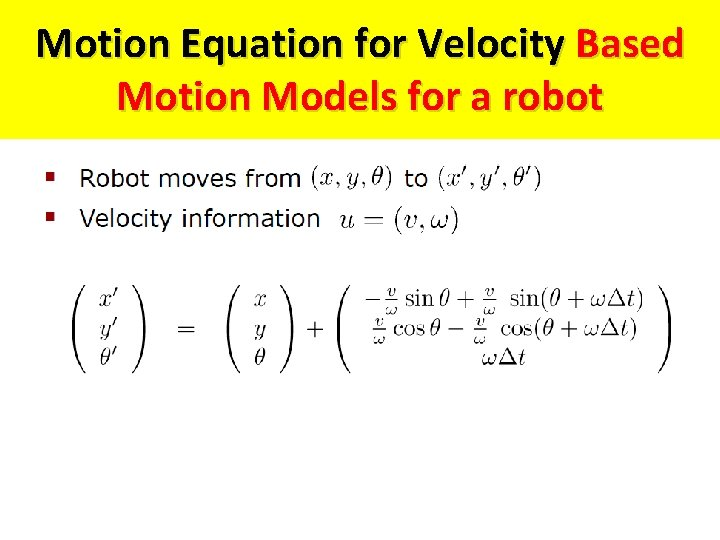Motion Equation for Velocity Based Motion Models for a robot• We add an additional noise term now.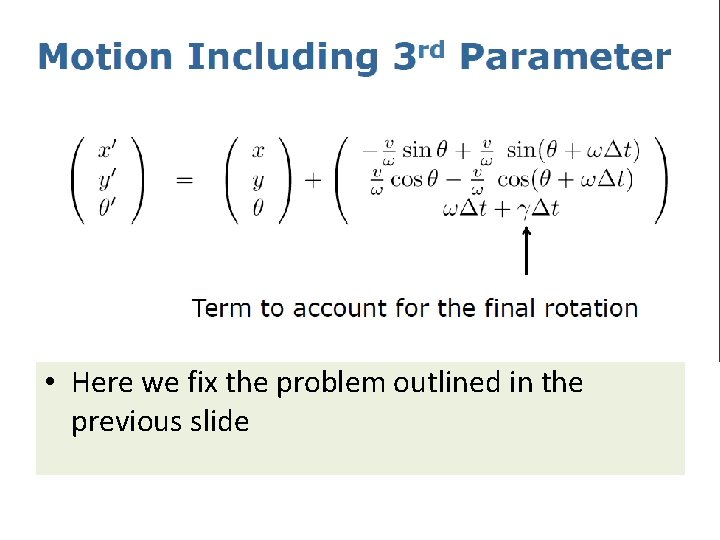• Here we fix the problem outlined in the previous slide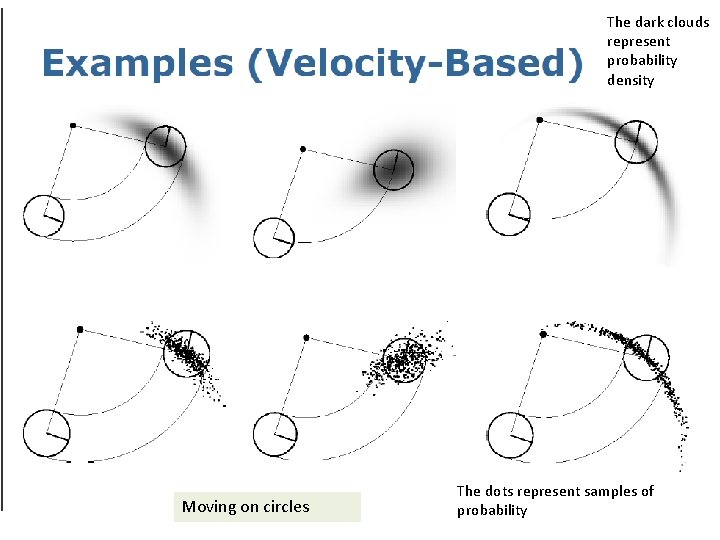The dark clouds represent probability density Moving on circles The dots represent samples of probabilitySensor Models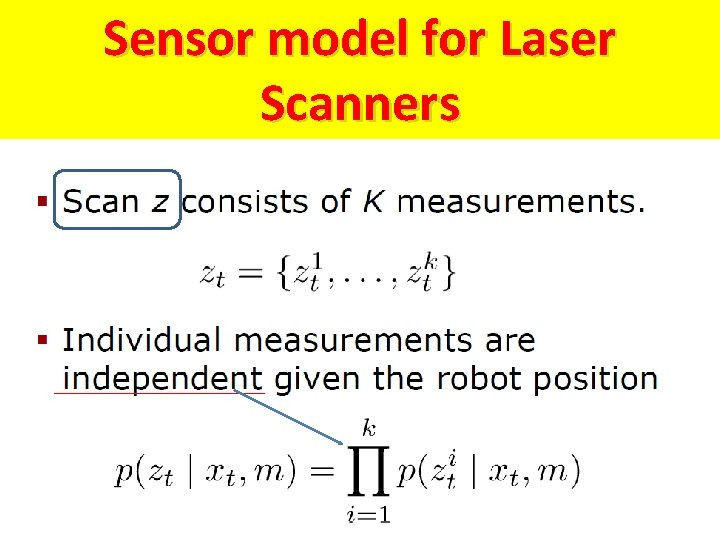Sensor model for Laser Scanners• You do not need to know too much if you are small and only want to move straight ahead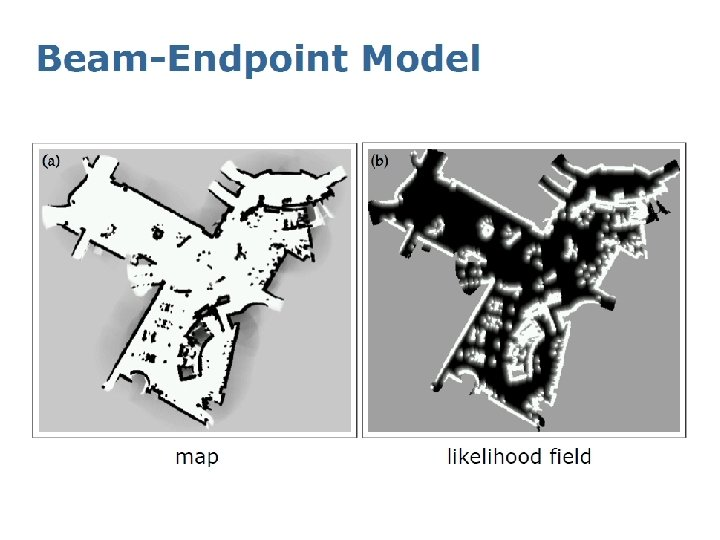Ray Cast Sensor ModelFeature-based Model for Range-Bearing Sensors errorSummary • Bayes Filter is a framework for state estimation • Motion model and sensor model are the central models in Bayes Filter • These are all standard models for: – Robot motion – Laser-based range sensing – Similar sensors